# Sputnik

The first Earth satellite was flying at speed 8000 m/s. At that rate he circled the earth in 82 minutes. Jet flies at an average speed 800 km/h. How long would it take circle the earth round?

t =  49.2 h

### Step-by-step explanation: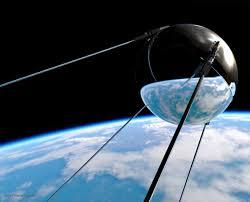Did you find an error or inaccuracy? Feel free to write us. Thank you!Tips to related online calculators
Check out our ratio calculator.
Do you want to convert velocity (speed) units?
Do you want to convert time units like minutes to seconds?

## Related math problems and questions:

• Earth's diameter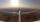The Earth's diameter on the equator is approximately 12750 km. How long does the Gripen fly over the Earth above the equator at 10 km if it is at an average speed of 1500 km / h?
• Two airportsTwo airports are 2690 km away. From the first airport, the airplane flies at a speed of 600 km/h, from a second airplane at a speed of 780 km/h. When will they meet if they flew at 10:00? How far from the first airport?
• Reconstruction of the corridorCalculate how many minutes will be reduced to travel 187 km long railway corridor, where the maximum speed increases from 120 km/h to 160 km/h. Calculate how many minutes will shorten travel time, if we consider that the train must stop at 6 stations, eac
• Two aircraftTwo planes fly to the airport. At some point, the first airplane is away from the airport 98 km and the second 138 km. The first aircraft flies at an average speed of 420 km/h, the second average speed is 360 km/h, while the tracks of both planes are perp
• Average speed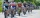The average speed of a pedestrian who walked 10 km was 5km/h, the average speed of a cyclist on the same track was 20km/h. In how many minutes did the route take more than a cyclist? Q
• Two planes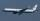Two planes are flying from airports A and B, 420 km distant from each other. The plane from A took off 15 minutes later and flies at an average speed of 40 km/h higher than the plane from B. Determine the average speed of these two aircraft if you know th
• On the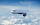On the map of Europe made at 1: 4000000, Bratislava and Paris' distance is 28 cm. At what time an airplane flying 800 km/h will fly this journey?
• Motion problemFrom Levíc to Košíc go car at speed 81 km/h. From Košíc to Levíc go another car at speed 69 km/h. How many minutes before the meeting will be cars 27 km away?
• Average speed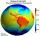What is the average speed you have to move the way around the world in 80 days? (Path along the equator, round to km/h).
• Two citiesCities A and B are 200 km away. At 7 o'clock from city A, the car started at an average speed of 80 km/h, and from B at 45 min later, the motorcycle is started at an average speed of 120 km/h. How long will they meet, and at what distance from point A wil
• MotionFrom two different locations distant 232 km started against car and bus. The car started at 5:20 with average speed 64 km/h. Bus started at 7:30 with average speed 80 km/h. When they meet? How many kilometers went the bus?
• Trains for people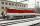It is said that the train is synonymous to delay. Calculate the average speed of travel by train long 85 km, with regular train leave at 7:00 and arrive at 8:18, but train is late and has departure at 8:10 and arrive at 9:27.
• GrandmotherMom walked out to visit her grandmother in a neighboring village 5km away and moved at a speed of 4km/h. An hour later, father drove down the same road at an average speed of 64km/h. 1) How long will take to catch mom die? 2) What is the approximate dista
• Earth and Sun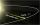Earth is 150 million km away from the Sun. 300,000 km of sunlight travels in 1 second. How long will the light come from the Sun to the Earth?
• Sound speed in Earth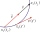Through the surface layers of the Earth, sound propagates 13 times faster than through air. During the geological survey, the charge exploded 1.7 km from the measuring station. How long does it take between the vibration of the soil at the measuring point
• Cars motion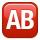From point A car start at the speed of 90 km/h. Against it departs from the point B at the same time another car at 60 km/h. For how long and where they met if the distance between A and B is 180 km?
• Two aircraftFrom the airport will start simultaneously two planes, which fly tracks are perpendicular to each other. The first flying speed of 680 km/h and the second 840 km/h. Calculate how far the aircraft will fly for half an hour.